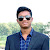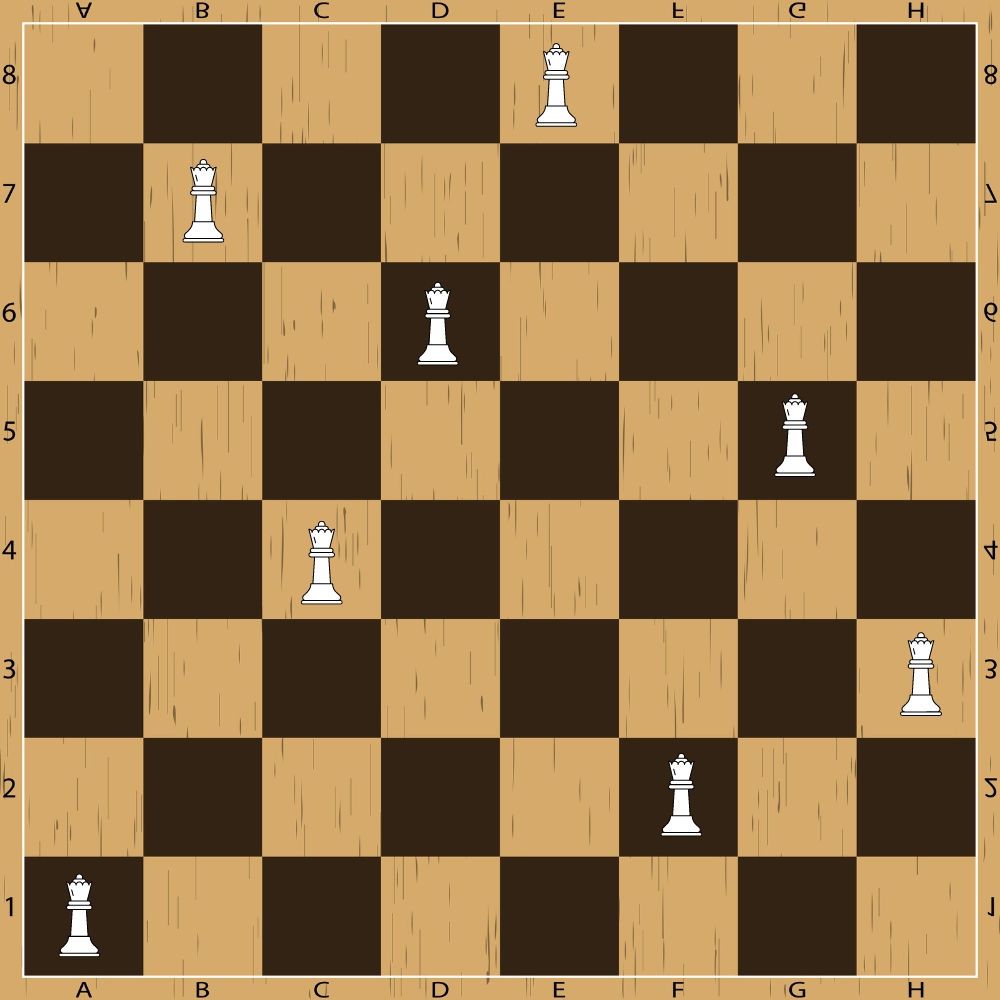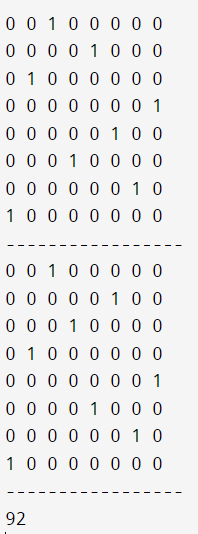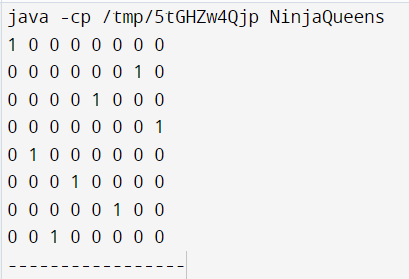New update is available. Click here to update.
Last Updated: Nov 1, 2023
Hard

# 8 Queen Problem Using BacktrackingManish Kumar
1 upvote

## Introduction

Backtracking is a recursive approach for solving any problem where we must search among all the possible solutions following some constraints. More precisely, we can say that it is an improvement over the brute force technique. In this blog, we will learn one popular DSA question: 8 queens problem using Backtracking.

We will retain the backtracking approach and go through its time optimization. Let's start with the problem statement of solving 8 queens problem using backtracking in the next section.

## Problem Statement

Given an 8x8 chess board, you must place 8 queens on the board so that no two queens attack each other. Print all possible matrices satisfying the conditions with positions with queens marked with '1' and empty spaces with '0'. You must solve the 8 queens problem using backtracking.

• Note 1: A queen can move vertically, horizontally and diagonally in any number of steps.
• Note 2: You can also go through the N-Queen Problem for the general approach to solving this problem.

### Sample Example

Example: One possible solution to the 8 queens problem using backtracking is shown below. In the first row, the queen is at E8 square, so we have to make sure no queen is in column E and row 8 and also along its diagonals. Similarly, for the second row, the queen is on the B7 square, thus, we have to secure its horizontal, vertical, and diagonal squares. The same pattern is followed for the rest of the queens.Output:

0 0 0 0 1 0 0 0

0 1 0 0 0 0 0 0

0 0 0 1 0 0 0 0

0 0 0 0 0 0 1 0

0 0 1 0 0 0 0 0

0 0 0 0 0 0 0 1

0 0 0 0 0 1 0 0

1 0 0 0 0 0 0 0

## Bruteforce Approach

One brute-force approach to solving this problem is as follows:

• Generate all possible permutations of the numbers 1 to 8, representing the columns on the chessboard.
• For each permutation, check if it represents a valid solution by checking that no two queens are in the same row or diagonal.
• If a valid solution is found, print the board layout.

While this approach works for small numbers, it quickly becomes inefficient for larger sizes as the number of permutations to check grows exponentially. More efficient algorithms, such as backtracking or genetic algorithms, can be used to solve the problem in a more optimized way.

## Backtracking Approach

This approach rejects all further moves if the solution is declined at any step, goes back to the previous step and explores other options.

### Algorithm

Let's go through the steps below to understand how this algorithm of solving the 8 queens problem using backtracking works:

• Step 1: Traverse all the rows in one column at a time and try to place the queen in that position.
• Step 2: After coming to a new square in the left column, traverse to its left horizontal direction to see if any queen is already placed in that row or not. If a queen is found, then move to other rows to search for a possible position for the queen.
• Step 3: Like step 2, check the upper and lower left diagonals. We do not check the right side because it's impossible to find a queen on that side of the board yet.
• Step 4: If the process succeeds, i.e. a queen is not found, mark the position as '1' and move ahead.
• Step 5: Recursively use the above-listed steps to reach the last column. Print the solution matrix if a queen is successfully placed in the last column.
• Step 6: Backtrack to find other solutions after printing one possible solution.

### Implementation in C++

Let's go through the C++ implementation of the above-discussed approach to solve the 8 queens problem using backtracking.

``````//Coding Ninjas
//C++ solution to 8 queens problem using backtracking
#include <bits/stdc++.h>
using namespace std;
int countt=0;

// A function to print a solution
void print(int board[]){
for(int i=0;i<8;i++){
for(int j=0;j<8;j++){
cout<<board[i][j]<<" ";
}
cout<<endl;
}
cout<<"-----------------\n";
}

//Function to check whether a position is valid or not
bool isValid(int board[],int row,int col){

//loop to check horizontal positions
for(int i=col;i>=0;i--){
if(board[row][i])
return false;
}
int i=row,j=col;

//loop to check the upper left diagonal
while(i>=0&&j>=0){
if(board[i][j])
return false;
i--;
j--;
}
i=row;
j=col;

//loop to check the lower left diagonal
while(i<8&&j>=0){
if(board[i][j])
return false;
i++;
j--;
}
return true;
}

//function to check all the possible solutions
void ninjaQueens(int board[],int currentColumn){
if(currentColumn>=8)
return;
//loop to cover all the columns
for(int i=0;i<8;i++){
if(isValid(board,i,currentColumn)){
board[i][currentColumn]=1;
if(currentColumn==7){
print(board);
countt++;
}
//recursively calling the function
ninjaQueens(board,currentColumn+1);
//backtracking
board[i][currentColumn]=0;
}

}
}
int main() {

//initial board situation
int board={{0,0,0,0,0,0,0,0},
{0,0,0,0,0,0,0,0},
{0,0,0,0,0,0,0,0},
{0,0,0,0,0,0,0,0},
{0,0,0,0,0,0,0,0},
{0,0,0,0,0,0,0,0},
{0,0,0,0,0,0,0,0},
{0,0,0,0,0,0,0,0}};
ninjaQueens(board,0);
/* In total, 92 solutions exist for 8x8 board. This statement will verify our code*/
cout<<countt<<endl;
return 0;
}``````

Output: (A total of 92 solutions will exist, we are showing the last two along with the count)### Implementation in Java

Let us look at the Java code for the algorithm implementation.

``````//Solution to 8 queens problem using backtracking
class NinjaQueens {

int countt=0;

// A function to print a solution
void print(int board[][]){
for(int i=0;i<8;i++){
for(int j=0;j<8;j++){
System.out.print(board[i][j]+" ");
}
System.out.print("\n");
}
System.out.print("-----------------\n");
}

//Function to check whether a position is valid or not
boolean isValid(int board[][],int row,int col){

//loop to check horizontal positions
for(int i=col;i>=0;i--){
if(board[row][i]==1)
return false;
}
int i=row,j=col;

//loop to check the upper left diagonal
while(i>=0&&j>=0){
if(board[i][j]==1)
return false;
i--;
j--;
}
i=row;
j=col;

//loop to check the lower left diagonal
while(i<8&&j>=0){
if(board[i][j]==1)
return false;
i++;
j--;
}
return true;
}

//Function to check all the possible solutions
void ninjaQueens(int board[][],int currentColumn){
if(currentColumn>=8)
return;
//loop to cover all the columns
for(int i=0;i<8;i++){
if(isValid(board,i,currentColumn)){
board[i][currentColumn]=1;
if(currentColumn==7){
print(board);
countt++;
}
//recursively calling the function
ninjaQueens(board,currentColumn+1);
//backtracking
board[i][currentColumn]=0;
}

}
}

void solve(){
//initial board situation
int board[][]={{0,0,0,0,0,0,0,0},
{0,0,0,0,0,0,0,0},
{0,0,0,0,0,0,0,0},
{0,0,0,0,0,0,0,0},
{0,0,0,0,0,0,0,0},
{0,0,0,0,0,0,0,0},
{0,0,0,0,0,0,0,0},
{0,0,0,0,0,0,0,0}};
ninjaQueens(board,0);
/* In total, 92 solutions exist for 8x8 board. This statement will verify our code*/
System.out.print("countt\n");
return;
}
public static void main(String[] args) {
NinjaQueens solution=new NinjaQueens();
solution.solve();
}
}``````

Output: The first solution is shown below.### Complexity Analysis

Time Complexity: O(N!), For the first column we will have N choices, then for the next column, we will have N-1 choices, and so on. Therefore the total time taken will be N*(N-1)*(N-2)...., which makes the time complexity to be O(N!).

Space Complexity: O(N^2), we can see that the initial space needed is N^2, then N^2-1, then N^2-2 and so on. Thus making the space complexity N^2N, but we don’t need all the N^2 options each time. Therefore, the overall space complexity is O(N^2).

### Implementation in Python

``````def is_valid(board, row, col, n):
# Check if no other queen is present in the same row or diagonal
for i in range(col):
if board[i] == row or \
board[i] - i == row - col or \
board[i] + i == row + col:
return False
return True

def solve_queen(board, col, n):
# Base case: if all queens are placed, return True
if col == n:
return True

# Try placing the queen in each row of the current column
for row in range(n):
if is_valid(board, row, col, n):
board[col] = row
# Recursively place the queens in the remaining columns
if solve_queen(board, col + 1, n):
return True
# Backtrack by undoing the current move and trying the next row
board[col] = -1

# If no valid position is found, return False
return False

def print_board(board, n):
for row in range(n):
for col in range(n):
if board[col] == row:
print('Q', end=' ')
else:
print('.', end=' ')
print()

def solve_n_queens(n):
board = [-1] * n
if solve_queen(board, 0, n):
print_board(board, n)
else:
print('No solution found')``````

The is_valid function checks if it is safe to place a queen in a given row and column by checking that no other queen is present in the same row or diagonal. The solve_queen function recursively tries to place the queens in each column and backtracks if no valid position is found. Finally, the print_board function prints the board layout for a valid solution, and the solve_n_queens function initializes the board and starts the recursive solving process.

## Optimising Time Complexity

We can optimize the 'isValid' Function and avoid checking the diagonals. The logic behind this idea is that the difference between row and column indices is constant for each diagonal, and the sum is constant for anti-diagonal.

### Algorithm

• Step 1: Create a boolean array to remember which diagonal is used by now.
• Step 2: In the 'isValid' Function, check if the boolean arrays are marked true instead of checking the diagonals.
• Step 3: Return true or false accordingly.

### Implementation in C++

``````//Coding Ninjas
//C++ solution to 8 queens problem using backtracking
#include <bits/stdc++.h>
using namespace std;
int countt=0;

//Using three boolean arrays to optimise the isValid function
bool leftDiagonal={0};
bool rightDiagonal={0};
bool column={0};

// A function to print a solution
void print(int board[]){
for(int i=0;i<8;i++){
for(int j=0;j<8;j++){
cout<<board[i][j]<<" ";
}
cout<<endl;
}
cout<<"-----------------\n";
}

//Function to check whether a position is valid or not
bool isValid(int board[],int row,int col){
if(leftDiagonal[row-col+7]!=1&&rightDiagonal[row+col]!=1&&column[row]!=1)
return true;
return false;
}

//function to check all the possible solutions
void ninjaQueens(int board[],int currentColumn){
if(currentColumn>=8)
return;
//loop to cover all the columns
for(int i=0;i<8;i++){
if(isValid(board,i,currentColumn)){
board[i][currentColumn]=1;
leftDiagonal[i-currentColumn+7]=rightDiagonal[i+currentColumn]=column[i]=1;
if(currentColumn==7){
print(board);
countt++;
}
//recursively calling the function
ninjaQueens(board,currentColumn+1);
//backtracking
board[i][currentColumn]=0;
leftDiagonal[i-currentColumn+7]=rightDiagonal[i+currentColumn]=column[i]=0;
}

}
}
int main() {

//initial board situation
int board={{0,0,0,0,0,0,0,0},
{0,0,0,0,0,0,0,0},
{0,0,0,0,0,0,0,0},
{0,0,0,0,0,0,0,0},
{0,0,0,0,0,0,0,0},
{0,0,0,0,0,0,0,0},
{0,0,0,0,0,0,0,0},
{0,0,0,0,0,0,0,0}};
ninjaQueens(board,0);
/* In total, 92 solutions exist for 8x8 board. This statement will verify our code*/
cout<<countt<<endl;
return 0;
}``````

Output:### Implementation in Java

``````//Solution to 8 queens problem using backtracking
class NinjaQueens {

int countt=0;

boolean[] leftDiagonal=new boolean;
boolean[] rightDiagonal=new boolean;
boolean[] column=new boolean;
// A function to print a solution

void print(int board[][]){
for(int i=0;i<8;i++){
for(int j=0;j<8;j++){
System.out.print(board[i][j]+" ");
}
System.out.print("\n");
}
System.out.print("-----------------\n");
}

//Function to check whether a position is valid or not
boolean isValid(int board[][],int row,int col){
if(leftDiagonal[row-col+7]==false&&rightDiagonal[row+col]==false&&column[row]==false)
return true;
return false;
}

//function to check all the possible solutions
void ninjaQueens(int board[][],int currentColumn){
if(currentColumn>=8)
return;
//loop to cover all the columns
for(int i=0;i<8;i++){
if(isValid(board,i,currentColumn)){
board[i][currentColumn]=1;
leftDiagonal[i-currentColumn+7]=rightDiagonal[i+currentColumn]=column[i]=true;
if(currentColumn==7){
print(board);
countt++;
}
//recursively calling the function
ninjaQueens(board,currentColumn+1);
//backtracking
board[i][currentColumn]=0;
leftDiagonal[i-currentColumn+7]=rightDiagonal[i+currentColumn]=column[i]=false;
}

}
}

void solve(){
//initial board situation
int board[][]={{0,0,0,0,0,0,0,0},
{0,0,0,0,0,0,0,0},
{0,0,0,0,0,0,0,0},
{0,0,0,0,0,0,0,0},
{0,0,0,0,0,0,0,0},
{0,0,0,0,0,0,0,0},
{0,0,0,0,0,0,0,0},
{0,0,0,0,0,0,0,0}};
ninjaQueens(board,0);
/* In total, 92 solutions exist for 8x8 board. This statement will verify our code*/
System.out.print("countt\n");
return;
}
public static void main(String[] args) {
NinjaQueens solution=new NinjaQueens();
solution.solve();
}
}``````

Output:### Complexity Analysis

Time Complexity: O(N!), For the first column, we will have N choices, then for the next column, we will have N-1 choices, and so on. Therefore the total time taken will be N*(N-1)*(N-2)...., which makes the time complexity to be O(N!).

Space Complexity: O(N), since we are just maintaining three linear arrays as extra storage space.

### What is 8 queen problem with example?

The 8 Queen Problem is a puzzle in which 8 queens must be placed on an 8x8 chessboard so that no two queens threaten each other. It is a classic problem in computer science and mathematics.

### How do you solve 8 queens problem backtracking?

To solve the 8 Queens Problem using backtracking, we recursively try to place queens in each column, checking if the placement is valid and backtracking if not. The process continues until a valid solution is found or no solution exists.

### Which algorithm is used to solve 8 queens problem?

One common algorithm used to solve the 8 Queens Problem is the backtracking algorithm, which tries to place queens on the chessboard column by column, checking if each placement is valid and backtracking if it is not.

### What is the logic behind Queens problem?

The N-Queens problem is a classic puzzle. Its logic involves placing N chess queens on an N×N chessboard, so that no two queens threaten each other. It's a constraint satisfaction problem commonly solved using backtracking algorithms.

### How many nodes are there in the 8 queen problem?

In the standard 8-Queens problem, there are 92 distinct solutions. Each solution represents a unique arrangement of 8 queens on an 8x8 chessboard, ensuring no two queens threaten each other in any direction.

## Conclusion

We extensively discussed solving the 8 queens problem using backtracking. We learned how this solution works, its output values and its optimisation. We also went through sample codes involving the solution of 8 queens problem using backtracking. You can also try the N-Queens problem at Coding Ninjas Studio.

We hope this blog has helped you enhance your knowledge about the topic 8 queens problem using backtracking. If you like to learn more, you can check out our articles: